# Basic Constructions

### From DT Online

##### Description

Although modern drafting aids and Computer Aided Design tools now do much of the work for us, an understanding of some basic Geometrical Construction can be useful when setting out large shapes, lofting or working on site such as on a stage set for example.

Typically, the equipment used in these constructions include: straight-edges and rules, set-squares, compasses, dividers and trammels or beam-compass.

Note: for large-scale work such as setting out foundations for a building or garden design, a beam-compass can be substituted by a peg and length of string, or a strip of wood with nails, and right angles can be constructed from triangles as described below.

##### Constructions
 Constructing a Right Angle using Triangles The Pythagoras Theorem states that ‘the square of the hypotenuse (the side opposite the right angle) is equal to the sum of the squares of the other two sides’. There are two particular right-angled triangles in which this relationship results in all sides being whole numbers and therefore easy to construct, using trammels for example. They are: the 3-4-5 Triangle (32 + 42 = 52) and the 5-12-13 Triangle (52 + 122 = 132) Draw which one of these best suits the work in hand to construct a right angle. ``` ```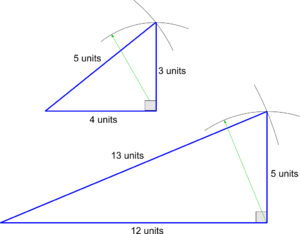Dividing a Line into Equal Parts Draw a construction line at an angle from the end of the line to be divided. Set dividers to any convenient size and step off the required number of equal divisions along this construction line. Join the last division on the construction line to the end of the line to be divided. Draw lines parallel to it through each of the other points. By Similar Triangles the original line is now divided into equal parts.Drawing Parallel Lines using Set-Squares and Straight-Edge Taking Dividing a Line into Equal Parts as an example, hold a set-square along the line joining the last division on the construction line to the end of the scale. Hold another set-square or a straight-edge along the first set-square as shown. Keeping the second set-square still, slide the first set-square along it to draw parallel lines through all the other points. ``` ```Constructing a 60 deg Angle This construction exploits the fact that the radius of a circle can be stepped round its circumference 6 times. Draw the arc of a circle of any convenient size, from a point on a line such that it intersects the line as shown. Without altering the compasses or dividers, step off the same radius along the arc from its intersection with the line. Join the point on the line to where this second arc intersects the first to give an angle of 60 deg. Note: Bisecting this angle gives 30 deg and bisecting again will provide 15 deg (see below).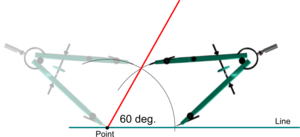Constructing a 90 deg Angle Start as for Constructing a 60 deg Angle but step off 2 radii along the circumference. From each of these two intersections on the circumference, strike two more arcs as shown. Join the intersection of these two arcs to the point on the line to construct a 90 deg right-angle. Note: Bisecting this angle gives 45 deg. (see below).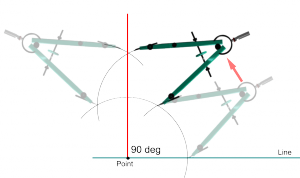Bisecting and Angle Strike an arc of any convenient radius from the apex of the angle to be bisected. From where this first arc intersects the two lines containing the angle, strike two more arcs to intersect with each other as shown. Join this new intersection to the apex of the angle to bisect it. Note: For lines at an angle but which do not intersect at a convenient apex, construct lines parallel to them and inside the angle to generate a apex which can be used. ``` ```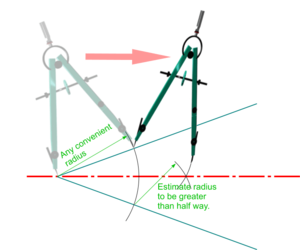Bisecting a Line From each end of the line to be bisected, strike arcs estimated to go beyond half way. Join the intersections of these two arcs to construct a second line at 90 deg to the first as shown. Note: For lines too long for this to be practical, arcs or successive arcs can be struck from each end to define a shorter length within which the centre must lie. ``` ```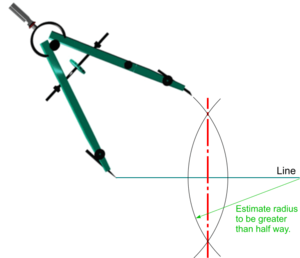Constructing Parallel Lines Strike arcs of the same radius from two widely spaced points on a line. Draw a line to touch the top of these two arcs to construct a second line parallel to the first. Note: The line drawn is a Tangent to the two arcs, so a more accurate method would be first to construct two lines perpendicular to the two points. These would be Normals to the Tangents and their intersections with the arcs would more clearly define where the parallel lines should touch the top of the arcs.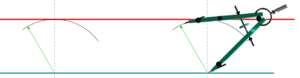Constructing a Perpendicular to Point on a Line With compasses or dividers set to any convenient distance, and with centre on the point, strike arcs to intersect the line on either side as shown. Use a similar construction to that for Bisecting a Line to create an intersection above the line (or above and below as a check on accuracy). Join the intersection(s) to the point to construct the perpendicularConstructing a Perpendicular from a Point to a Line From the given point above the line, strike arcs to intersect the line as shown. Use these two intersections and repeat the construction as for Constructing a Perpendicular to Point on a Line Join the point to the new intersections to construct the perpendicular.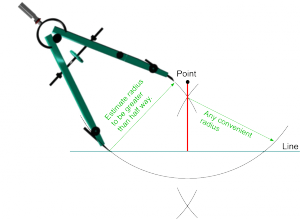Note: See the sections on Circles and Polygons for basic constructions relating to these shapes.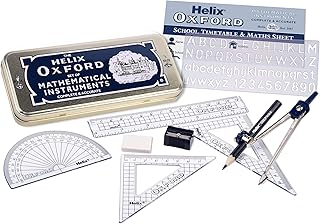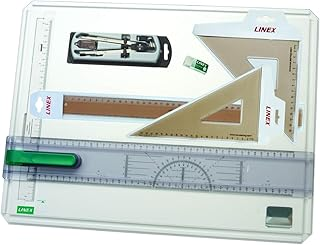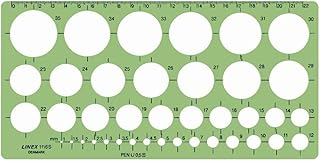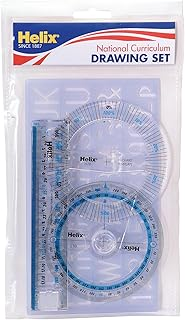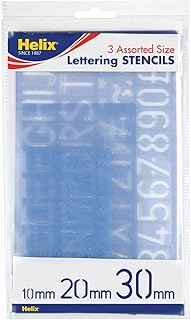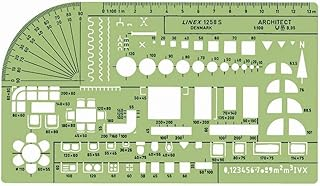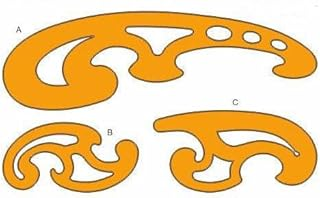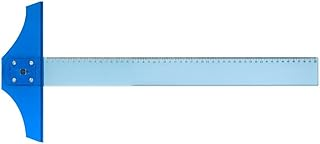Helix Oxford Maths SetLinex Student Saver BundleHelix Precision Plus Drawing SetPrimary Shape Template SetLinex Circle TemplateHelix National Curriculum Drawing SetHelix 3 Piece Stencil SetLinex Universal Architects TemplateFrench Curve Transparent TemplateT-Square